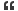# Thread: Digit sum

1. ##Digit sum

What is the sum of the digits of the decimal form of the product below?

1999 2001
2 x 5Reply With Quote

2. ## Re: Digit sum

Hi,

Your problem is not clear. what you want actually? what is the meaning of the following...

1999 2001
2 x 5

------------------
sureshReply With Quote

3. ## Re: Digit sum

I think you need the solution for the following....

(2^1999 * 5^2001) Sum of the results of this product...

Am i correct?

----------------
sureshReply With Quote

4. ## Re: Digit sum

Yes. You R CorrectReply With Quote

5. ## Re: Digit sum

The answer for your problem is 7.

Solution:

Your problem is (2^n * 5^(n+2))

Let's take n=1
result is 2*125 = 250 = 7

Let's take n=2
result is 4*625 = 2500 = 7

Let's take n=3
result is 8*3125 = 25000 = 7

Let's take n=4
result is 16*15625 = 250000 = 7
.
.
.
So finally we got the result like 250...... and the sum is 7

---------------------
sureshReply With Quote

6. ## Re: Digit sum

That's a Great Logic!
Thnks.
2^n*5^(n+2)
= 2^n*5^n*5^2
=(2*5)^n*25
=10^n*25
Sum is always 7.Reply With Quote

7. ## Re: Digit sum

great thinking suresh..Reply With Quote

8. ## Re: Digit sum

2^1999=(2^2000)/2

5^2001=(5^2000)*5

lhs={(2*5)^2000}*5/2=(10^2)^1000*2.5=(100^1000)*2.5=(1 followed by 20 zeroes)*2.5=25 followed by 19 zeroes=>this gives sum of digits=2+5=7Reply With Quote

9. ##Re: Digit sum

2^1999=(2^2000)/2

5^2001=(5^2000)*5

lhs={(2*5)^2000}*5/2

(10^2000)*2.5
{(10^2)^1000}*2.5

(100^1000)*2.5
(1 followed by 20 zeroes)*2.5

25 followed by 19 zeroes

hence, sum of digits =2+5=7

just getting the advantage of particular set of 2 & 5Reply With Quote

10. ## Re: Digit sumOriginally Posted by rahul149042^1999=(2^2000)/2

5^2001=(5^2000)*5

lhs={(2*5)^2000}*5/2

(10^2000)*2.5
{(10^2)^1000}*2.5

(100^1000)*2.5
(1 followed by 20 zeroes)*2.5

25 followed by 19 zeroes

hence, sum of digits =2+5=7

just getting the advantage of particular set of 2 & 5
Morning
I'm trying to do same problem guys
How did you get that:
(100^1000) = 1 followed by 20 zeros
Because what I got from 10^n
Letting n=1, 10
n=2, 100
.
.
.
n=10, 1 followed by 10 zerosReply With Quote

####Posting Permissions

• You may not post new threads
• You may not post replies
• You may not post attachments
• You may not edit your posts
•